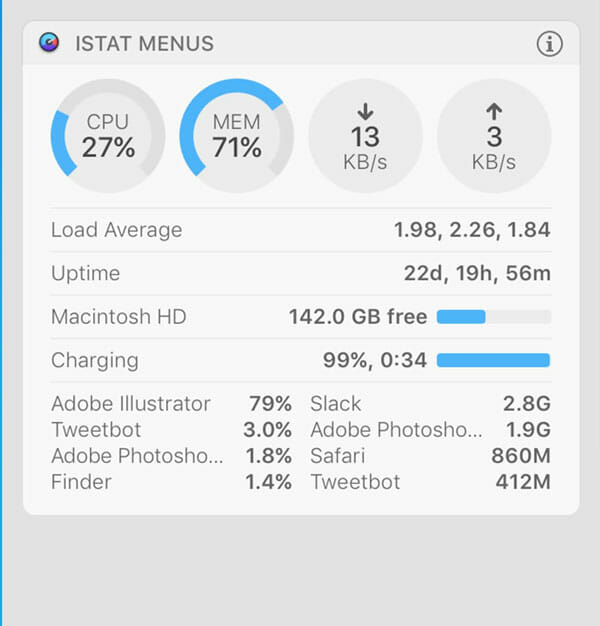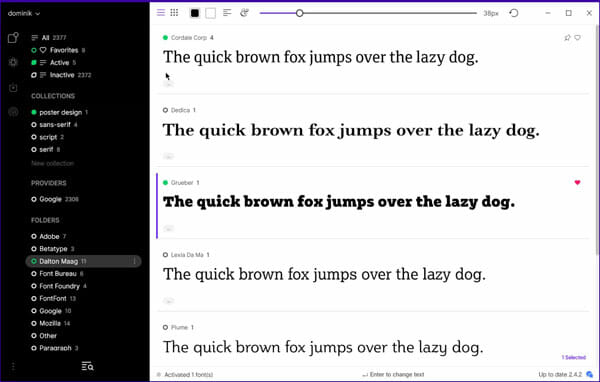### How To Use Windows Calculator For Number System Conversions

We use built-in Windows 7 / 8 Calculator to perform calculations like addition or subtraction. Some may use it to perform some complex operations to find trigonometric values, exponential values, percentages and more. But, do you know that you can even do number system conversions using Windows Calculator. You can convert decimal to binary, decimal to Octal, decimal to Hexadecimal and many more conversions like this using the built-in Windows Calculator.There is of installing the third party calculators to do such operations. You can use Windows Calculator to do these things, with just few clicks and we will see how to use Windows Calculator to do such conversions.

### How To Use Windows Calculator for Number System Conversions?

Windows Calculator is located at System 32 folder with the name as calc.exe and you can access it from the start search bar. There are basically four types of calculators in Windows by name Standard, Scientific, Programmer and Statistics.For this article to convert among number systems, we are going to use Programmer Calculator.

Type “calc” in the start search bar and hit enter. Calculator gets opened and we need to use the Programmer mode.

Click on “View” and you can see four modes as mentioned. Click on “Programmer” from the list and the calculator mode gets changed.In this Programmer mode, you can see four radio buttons for Hex (Hexadecimal), Dec (Decimal), Oct (Octal) and Bin (Binary). These are useful to convert number among numbering systems.

If you want to convert a number from decimal to binary, select the Dec radio button. Move to the Calculator screen and type a decimal number (say 1947).Now select the Bin radio button (as you want to convert decimal number to binary number). You can see that 1947 (decimal number) has been converted to 11110011011 (binary).If you want to convert the same decimal number to another number format, say Hexadecimal. Just click on the Hex radio button. That’s it!What you need to remember here is, if you want to convert a number from one format to another format (source format to destination format), first select the radio button of the source format.

Then enter the number and select the destination number format. In our example, we want to convert from decimal (source number format) to binary (destination number format). So, I selected Dec radio button, entered the number and selected Bin radio button.

### Conclusion

You can even use Windows Calculator to do date calculations, unit conversions and more. Converting from one number system to another is one of it’s feature and you can do a lot by exploring it. What interesting part have you done using Windows 7 / 8 Calculator? Please, do share with us through comments.

### Related articles### Most Useful Windows Apps For Students### Best CPU Temperature Monitoring Apps For Windows And Mac### How To Install And Use OpenSSH In Windows 10